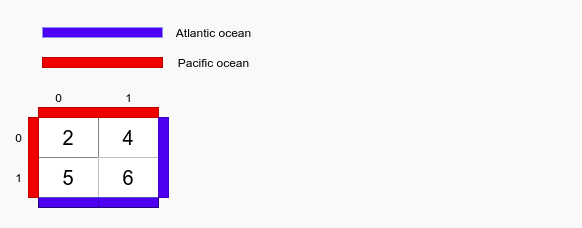# Water flow

Posted: 6 Jan, 2021
Difficulty: Hard

## PROBLEM STATEMENT

#### You need to find the list of coordinates from which water can flow to both the oceans. The list should be sorted in lexicographical order.##### Input Format:
``````The first line of the input contains an integer ‘T’ denoting the number of test cases.

The first line of each test case contains two space-separated positive integers ‘N’ and ‘M’ denoting the number of the rows and columns in a matrix 'A' respectively.

In the next ‘N’ lines of each test case, the ith line contains ‘M’ space-separated integers denoting the height of water.
``````
##### Output Format:
``````The first line of output of each test case should contain positive integer ‘K’ denoting the total number of coordinates.

The next ‘K’ lines contain one coordinate in each line.
``````
##### Note:
``````You do not need to print anything, it has already been taken care of. Just implement the given function.
``````
##### Constraints:
``````1 <= T <= 50
1 <= N <= 10^4
1 <= M <= 10^4
1 <= N*M <= 10^4
1 <= A[i][j] <= 10^9

Time Limit: 1 sec
``````Approach 1
• We will run two nested loops to traverse each cell of the matrix.
• For each cell, we perform DFS in which we go to all adjacent neighbours having their height lower than the current cell.
• We take two boolean variables to mark the reachability of that cell to the ocean. If we somehow reach any of the ocean we mark the corresponding boolean variable to be true.
• In the end, if both of the boolean variables are true then it means this cell can reach both of the oceans hence we include this cell in our answer.